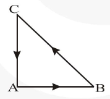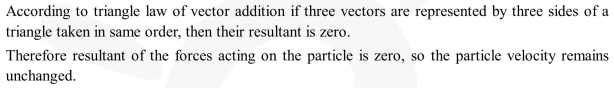Deepak Scored 45->99%ile with Bounce Back Crack Course. You can do it too!

# Three forces start acting

Question:

Three forces start acting simultaneously on a particle moving with velocity $\vec{v}$. These forces are represented in magnitude and direction by the three sides of a triangle $\mathrm{ABC}$ (as shown). The particle will now move with velocity-1. Less than $\vec{v}$

2. greater than $\vec{v}$

3. $|\mathrm{v}|$ in the direction of largest force $\mathrm{BC}$

4. $\vec{v}$, remaining unchanged

Correct Option: , 4

Solution: